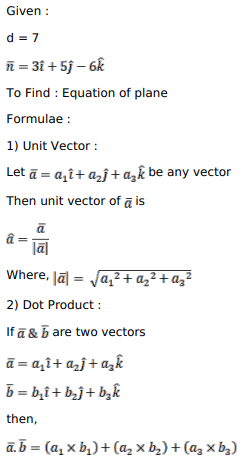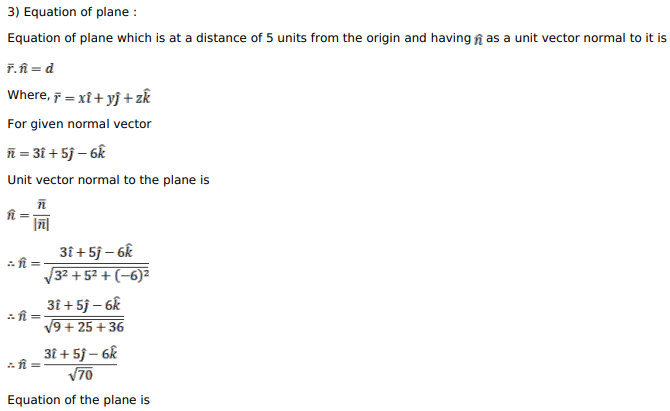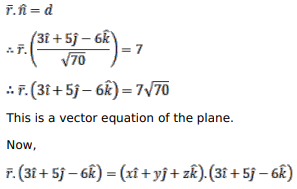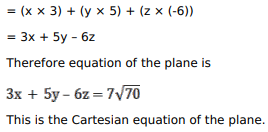# Find the vector and Cartesian equations of a plane which is at a distanceQuestion:

Find the vector and Cartesian equations of a plane which is at a distance of 7 units from the origin and whose normal vector from the origin is $(3 \hat{i}+5 \hat{j}-6 \hat{k})$.

Solution: